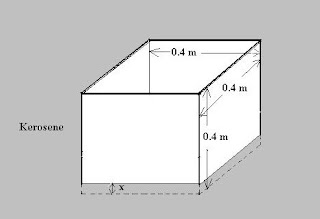## Pages

`“Life is like riding a bicycle.  To keep your balance you must keep moving.”–Albert Einstein`

## Friday, September 30, 2011

### AP Physics B - Free Response Practice Question on Fluid Mechanics

“Our greatest weakness lies in giving up. The most certain way to succeed is always to try just one more time.”

– Thomas A. Edison

Today I will give you a free response practice question on fluid mechanics. The question is meant for AP Physics B aspirants. But it will be useful for AP Physics C aspirants also as it high lights basic principles in hydrostatics. Generally questions meant for AP Physics C will be tougher than those for AP Physics B. So the question I give below can serve only as a part of a more difficult question in their case. Here is the question:The adjoining figure shows an empty thin walled cubical vessel of side 0.4 m and mass 6.4 kg floating on kerosene contained in a large tank (not shown). Density of kerosene is 800 kgm–3 where as the density of water is 1000 kgm–3. You may take the acceleration due to gravity as 10 ms–2. Now, answer the following questions:

(a) Calculate the magnitudes of the force of buoyancy and the force of gravity acting on the empty vessel and state their directions.

(b) Calculate the height x of the portion of the empty vessel that is submerged in kerosene.

(c) Water is slowly poured into the vessel so that an additional 0.2 m of the height of the vessel is submerged in kerosene. Calculate the volume of water added to obtain this condition.

Try to answer the above question which carries 10 points. You have about 11 minutes for answering it. I’ll be back shortly with a model answer for your benefit.

You will find a useful post in this section here.